• 导向滤波matlab代码相关合集，包含增强算法，羽化算法，滤波平滑算法等。导向滤波（Guided Filtering）和双边滤波（BF）、最小二乘滤波（WLS）是三大边缘保持（Edge-perserving）滤波器。当然，引导滤波的功能不仅仅...
• 导向滤波同样是一种平滑滤波算法，其与最小二乘滤波和双边滤波相比，同样是一种具有边缘保持的功能的图形滤波算法，可以用于处理图形噪点较多的图像，而且此种滤波算法与最小二乘滤波和双边滤波相比，有其独特的特点...
   导向滤波同样是一种平滑滤波算法，其与最小二乘滤波和双边滤波相比，同样是一种具有边缘保持的功能的图形滤波算法，可以用于处理图形噪点较多的图像，而且此种滤波算法与最小二乘滤波和双边滤波相比，有其独特的特点，让我们来首先简述一下其滤波的基本原理：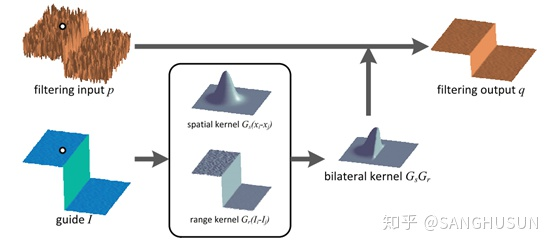滤波过程示意图  这是来源于Kaiming He（何恺明教授）其文章中的原理图，其中的p为输入图像，其中的q为输出图像，I为输入的引导图像，其中引导图像经过一个双边滤波核与输入图像进行结合，最终获得输出的图像。导向滤波其基本的算法原理可以见下图：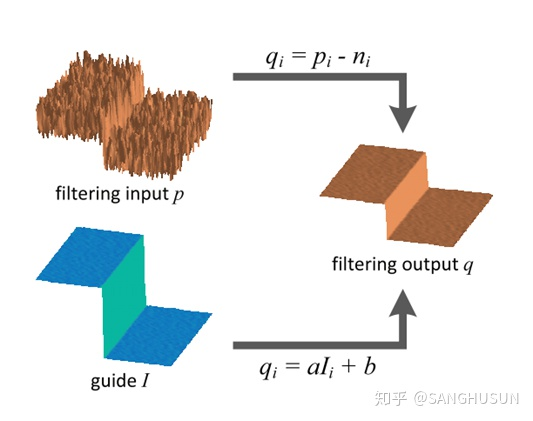算法原理其中输出图像q有：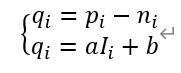其中的输出图像q又有如下表达：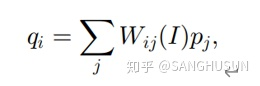我们可以看到的是Wij为权值，这个权值限定了图像q的输出，而这个权值的来源是引导图像I，所以W是一个与I有关的数值，且其为一个滤波核，其中i与j为图像的像素坐标引导，由此表达式我们可以直观的看出，输出图像和输入图像p，存在的是一种线性关系。 在导向滤波中，核心的假设在于，在一个以k为核心的处理窗口中，输出图像的q是一种线性的模式，也即：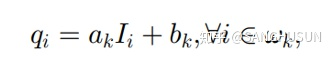其中的ak和bk为窗口中的常系数，同时我们需要设定一个正方形的窗格，此窗格的半径为r。同时ak和bk的来源，我们都通过上文得知，其由输入的图像来进行限制，我们认为，输出的图像是输入图像减去噪声而形成的，故有：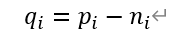其中的ni为图像中的噪声，而我们需要将此图像中的噪声降低到最低，所以需要找到一种比较好的方法来达到这一点：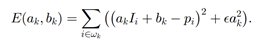我们可以看到上式为一个关于ak和bk的像素损失函数，同时我们又有一个调整参数，我们将调整参数设定为e，这个调整参数限定了ak。在何凯明教授之后的文中提到，此函数是一种脊回归模型，我们可以获取ak和bk其显式解为：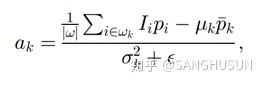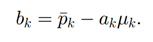其中uk为引导图I中的窗口中的像素均值，而为窗口中的像素方差，而下式的e则为调整参数，而w为窗口的像素点的个数，而下式中的pk为：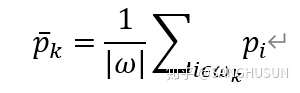那么当我们将此应用于具体的图像中时，对于一个N*N的处理窗格，一个像素点就会被包含N次，也即我们需要利用w，来进行取均值的处理：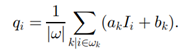同时我们注意到ak和bk，所以我们可以将上式改写为：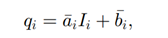这样我们就建立了一个，从p，再依据I，最后到q的一种映射的过程，这也就是导向滤波整体算法原理，而当我们的导向图I和输入图片为同一张图片时，此种滤波算法就具有边缘保持的能力。我们总结一下此种算法即：其输入端有4个输入：输入图像p，引导图像I，处理窗口半径r，调整参数e。而其输出为一张图像：输出图像q。其具体的算法实现过程：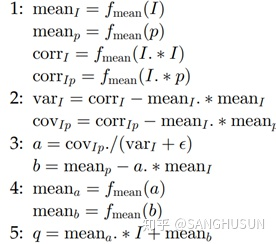我们利用matlab进行对原始的噪点较多的图形进行处理，首先我们是可以得知，此处我们输入图像和引导图像为同一张图像，此处我们设置三种窗口的规格r0=2;r1=3;r2=4；而对于调整参数，我们同样设置三种：Eps0=0.01，eps1=0.04，eps2=0.09；故对于此滤波器的调用方式为：subplot(3,3,1);gfout1_1=guidedfilter(gfI,gfp,r0,eps0);imshow(gfout1_1);title('r=2,eps=0.01');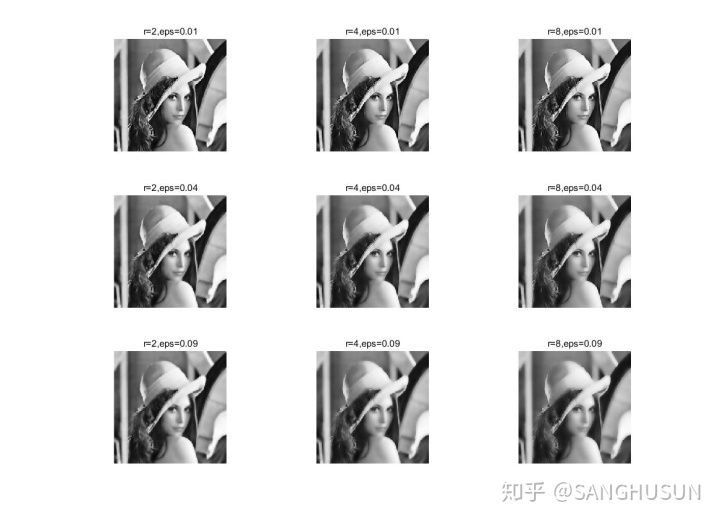lena女神Guidedfilter.h:
function q = guidedfilter(I, p, r, eps)

%   - guidance image: I (should be a gray-scale/single channel image)
%   - filtering input image: p (should be a gray-scale/single channel image)
%   - local window radius: r
%   - regularization parameter: eps

[hei, wid] = size(I);
N = boxfilter(ones(hei, wid), r);

mean_I = boxfilter(I, r) ./ N;
mean_p = boxfilter(p, r) ./ N;
mean_Ip = boxfilter(I.*p, r) ./ N;
% this is the covariance of (I, p) in each local patch.
cov_Ip = mean_Ip - mean_I .* mean_p;

mean_II = boxfilter(I.*I, r) ./ N;
var_I = mean_II - mean_I .* mean_I;

a = cov_Ip ./ (var_I + eps);
b = mean_p - a .* mean_I;

mean_a = boxfilter(a, r) ./ N;
mean_b = boxfilter(b, r) ./ N;

q = mean_a .* I + mean_b;
endboxfilter.h:
function imDst = boxfilter(imSrc, r)

%   BOXFILTER   O(1) time box filtering using cumulative sum
%
%   - Definition imDst(x, y)=sum(sum(imSrc(x-r:x+r,y-r:y+r)));
%   - Running time independent of r;
%   - Equivalent to the function: colfilt(imSrc, [2*r+1, 2*r+1], 'sliding', @sum);
%   - But much faster.

[hei, wid] = size(imSrc);
imDst = zeros(size(imSrc));

%cumulative sum over Y axis
imCum = cumsum(imSrc, 1);
%difference over Y axis
imDst(1:r+1, :) = imCum(1+r:2*r+1, :);
imDst(r+2:hei-r, :) = imCum(2*r+2:hei, :) - imCum(1:hei-2*r-1, :);
imDst(hei-r+1:hei, :) = repmat(imCum(hei, :), [r, 1]) - imCum(hei-2*r:hei-r-1, :);

%cumulative sum over X axis
imCum = cumsum(imDst, 2);
%difference over Y axis
imDst(:, 1:r+1) = imCum(:, 1+r:2*r+1);
imDst(:, r+2:wid-r) = imCum(:, 2*r+2:wid) - imCum(:, 1:wid-2*r-1);
imDst(:, wid-r+1:wid) = repmat(imCum(:, wid), [1, r]) - imCum(:, wid-2*r:wid-r-1);
end算法总体评价：导向滤波作为三大边缘保持滤波器之一，其平滑效果和边缘保持能力值得肯定，但是其同时因为其算法原因会产生光晕（halo）此点何恺明教授也在其文章中详细说明，其与另外两种边缘保持滤波算法相比，其一大特点就在于其输入，其输入为一张输入图像和一张引导图像，所以这点是一个非常鲜明的特点，同时需注意的是，要是其存在边缘保持的滤波效果，需要使引导图像和输入图像为同一张图像，而且其处理窗口和损失参数的设置对其滤波效果的影响较大，此点可详见上文。参考文献____________________________________________________________________________________________Guided Image Filtering, by Kaiming He, Jian Sun, and Xiaoou Tang, in TPAMI 2013.SANGHUSUN2020.02.10
展开全文• Kaiming He的导向滤波matlab代码。
• ## 导向滤波

千次阅读 2014-07-21 11:35:42
何凯明去雾算法中的导向滤波实现，原文地址导向滤波。 导向图像I，滤波输入图像p以及输出图像q。像素点 i 处的滤波结果是被表达成一个加权平均： 假设导向滤波器在导向图像I和滤波输出q之间是一个局部线性模型...

何凯明去雾算法中的导向滤波实现，原文地址导向滤波。
导向图像I，滤波输入图像p以及输出图像q。像素点 i 处的滤波结果是被表达成一个加权平均：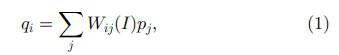假设导向滤波器在导向图像I和滤波输出q之间是一个局部线性模型：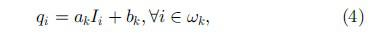最小化下面的窗口Wk的代价函数：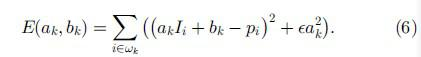用来确定a,b的值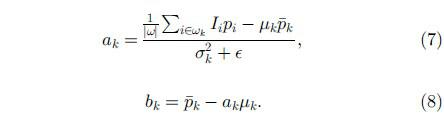其中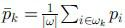论文所给算法如下：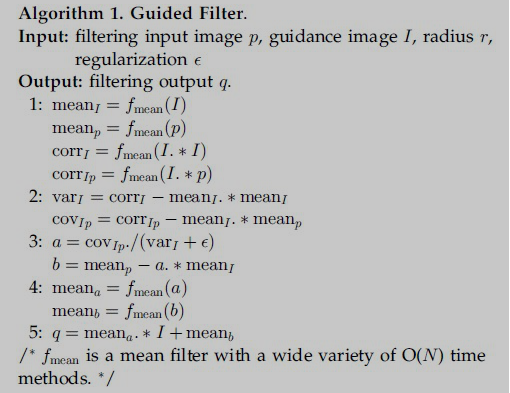matlab代码如下：

[plain] view
plaincopyfunction q = guidedfilter(I, p, r, eps)
%   GUIDEDFILTER   O(1) time implementation of guided filter.
%
%   - guidance image: I (should be a gray-scale/single channel image)
%   - filtering input image: p (should be a gray-scale/single channel image)
%   - local window radius: r
%   - regularization parameter: eps

[hei, wid] = size(I);
N = boxfilter(ones(hei, wid), r); % the size of each local patch; N=(2r+1)^2 except for boundary pixels.

mean_I = boxfilter(I, r) ./ N;
mean_p = boxfilter(p, r) ./ N;
mean_Ip = boxfilter(I.*p, r) ./ N;
cov_Ip = mean_Ip - mean_I .* mean_p; % this is the covariance of (I, p) in each local patch.

mean_II = boxfilter(I.*I, r) ./ N;
var_I = mean_II - mean_I .* mean_I;

a = cov_Ip ./ (var_I + eps); % Eqn. (5) in the paper;
b = mean_p - a .* mean_I; % Eqn. (6) in the paper;

mean_a = boxfilter(a, r) ./ N;
mean_b = boxfilter(b, r) ./ N;

q = mean_a .* I + mean_b; % Eqn. (8) in the paper;
end

[plain] view
plaincopyfunction imDst = boxfilter(imSrc, r)

%   BOXFILTER   O(1) time box filtering using cumulative sum
%
%   - Definition imDst(x, y)=sum(sum(imSrc(x-r:x+r,y-r:y+r)));
%   - Running time independent of r;
%   - Equivalent to the function: colfilt(imSrc, [2*r+1, 2*r+1], 'sliding', @sum);
%   - But much faster.

[hei, wid] = size(imSrc);
imDst = zeros(size(imSrc));

%cumulative sum over Y axis
imCum = cumsum(imSrc, 1);
%difference over Y axis
imDst(1:r+1, :) = imCum(1+r:2*r+1, :);
imDst(r+2:hei-r, :) = imCum(2*r+2:hei, :) - imCum(1:hei-2*r-1, :);
imDst(hei-r+1:hei, :) = repmat(imCum(hei, :), [r, 1]) - imCum(hei-2*r:hei-r-1, :);

%cumulative sum over X axis
imCum = cumsum(imDst, 2);
%difference over Y axis
imDst(:, 1:r+1) = imCum(:, 1+r:2*r+1);
imDst(:, r+2:wid-r) = imCum(:, 2*r+2:wid) - imCum(:, 1:wid-2*r-1);
imDst(:, wid-r+1:wid) = repmat(imCum(:, wid), [1, r]) - imCum(:, wid-2*r:wid-r-1);
end

以下为单通道图像导向滤波opencv实现：

[cpp] view
plaincopy#include "myGuidedFilter_Mat.h"

CvMat * cumsum(CvMat *src,int rc)
{
CvMat *Imdst = cvCreateMat(src->rows,src->cols,CV_64FC1);
Imdst=cvCloneMat(src);
if (rc==1)
{
for(int y=1;y<src->height;y++)
{
double *ptr0=(double *)(Imdst->data.ptr+(y-1)*Imdst->step);
double *ptr=(double *)(Imdst->data.ptr+y*Imdst->step);
for(int x=0;x<src->width;x++)
{
ptr[x]=ptr0[x]+ptr[x];
//cvSetReal2D(Imdst,y,x,cvGetReal2D(Imdst,y-1,x)+cvGetReal2D(Imdst,y,x));
}
}
}
else if (rc==2)
{
for(int y=0;y<src->height;y++)
{
double *ptr=(double *)(Imdst->data.ptr+y*Imdst->step);
for(int x=1;x<src->width;x++)
{
ptr[x]=ptr[x-1]+ptr[x];
//cvSetReal2D(Imdst,y,x,cvGetReal2D(Imdst,y,x-1)+cvGetReal2D(Imdst,y,x));
}
}
}
return Imdst;
}
CvMat * boxFilter(CvMat *src,int r)
{
CvMat *Imdst = cvCreateMat(src->rows,src->cols,CV_64FC1);
Imdst=cvCloneMat(src);
CvMat *subImage;

//imCum = cumsum(imSrc, 1);
CvMat *imCum = cumsum(Imdst,1);

//imDst(1:r+1, :) = imCum(1+r:2*r+1, :);
for (int y = 0;y<r;y++)
{
//double *ptrDst=(double *)Imdst->data.ptr+y*Imdst->step;
//double *ptrCum=(double *)imCum->data.ptr+(y+r)*imCum->step;
for(int x = 0;x<Imdst->width;x++)
{
//ptrDst[x]=ptrCum[x];
cvSetReal2D(Imdst,y,x,cvGetReal2D(imCum,y+r,x));
}
}

//imDst(r+2:hei-r, :) = imCum(2*r+2:hei, :) - imCum(1:hei-2*r-1, :);
for (int y = r+1;y<Imdst->height-r-1;y++)
{
for(int x = 0;x<Imdst->width;x++)
{
cvSetReal2D(Imdst,y,x,(cvGetReal2D(imCum,y+r,x)-cvGetReal2D(imCum,y-r-1,x)));
}
}

//imDst(hei-r+1:hei, :) = repmat(imCum(hei, :), [r, 1]) - imCum(hei-2*r:hei-r-1, :);
subImage = cvCreateMat(r,Imdst->width,CV_64FC1);
CvMat *tem=cvCreateMat(1,Imdst->width,CV_64FC1);
cvGetRow(imCum,tem,imCum->height-1);
cvRepeat(tem,subImage);
/*for(int y=0;y<r;y++)
{
for(int x=0;x<Imdst->width;x++)
{
cvSetReal2D(subImage,y,x,cvGetReal2D(imCum,Imdst->height-1,x));
}
}*/
for (int y = Imdst->height-r;y<Imdst->height;y++)
{
for(int x = 0;x<Imdst->width;x++)
{
cvSetReal2D(Imdst,y,x,cvGetReal2D(subImage,y-Imdst->height+r,x)-cvGetReal2D(imCum,y-r-1,x));
}
}
cvReleaseMat(&subImage);
cvReleaseMat(&tem);

imCum = cumsum(Imdst, 2);
//imDst(:, 1:r+1) = imCum(:, 1+r:2*r+1);
for (int y = 0;y<Imdst->height;y++)
{
for(int x = 0;x<r;x++)
{
cvSetReal2D(Imdst,y,x,cvGetReal2D(imCum,y,x+r));
}
}

//imDst(:, r+2:wid-r) = imCum(:, 2*r+2:wid) - imCum(:, 1:wid-2*r-1);
for (int y = 0;y<Imdst->height;y++)
{
for(int x = r+1;x<Imdst->width-r-1;x++)
{
cvSetReal2D(Imdst,y,x,(cvGetReal2D(imCum,y,x+r)-cvGetReal2D(imCum,y,x-r-1)));
}
}

//imDst(:, wid-r+1:wid) = repmat(imCum(:, wid), [1, r]) - imCum(:, wid-2*r:wid-r-1);
subImage = cvCreateMat(Imdst->height,r,CV_64FC1);
tem=cvCreateMat(Imdst->height,1,CV_64FC1);
cvGetCol(imCum,tem,imCum->width-1);
cvRepeat(tem,subImage);
/*for(int y=0;y<Imdst->height;y++)
{
for(int x=0;x<r;x++)
{
cvSetReal2D(subImage,y,x,cvGetReal2D(imCum,y,Imdst->width-1));
}
}*/
for (int y = 0;y<Imdst->height;y++)
{
for(int x = Imdst->width-r;x<Imdst->width;x++)
{
cvSetReal2D(Imdst,y,x,cvGetReal2D(subImage,y,x-Imdst->width+r)-cvGetReal2D(imCum,y,x-r-1));
}
}
cvReleaseMat(&subImage);

return Imdst;
}

CvMat * myGuidedFilter_Mat(CvMat * I,CvMat *img_pp,int r, double eps)
{
int height = img_pp->height;
int width = img_pp->width;
int type = CV_64FC1;

CvMat *ones = cvCreateMat(height,width,type);
cvSet(ones,cvRealScalar(1));
CvMat * N = boxFilter(ones,r);

//求I的均值
CvMat * mean_I = cvCreateMat(height,width,type);
cvDiv(boxFilter(I,r),N,mean_I);
//求P的均值
CvMat * mean_p = cvCreateMat(height,width,type);
cvDiv(boxFilter(img_pp,r),N,mean_p);
//求I*P的均值
CvMat * pr = cvCreateMat(height,width,type);
cvMul(I,img_pp,pr);
CvMat * mean_Ip = cvCreateMat(height,width,type);
cvDiv(boxFilter(pr,r),N,mean_Ip);

//求I与p协方差
cvMul(mean_I,mean_p,pr);
CvMat * cov_Ip = cvCreateMat(height,width,type);
cvSub(mean_Ip,pr,cov_Ip);

//求I的方差
CvMat * var_I  = cvCreateMat(height,width,type);
cvMul(I,I,pr);
cvDiv(boxFilter(pr,r),N,var_I);
cvMul(mean_I,mean_I,pr);
cvSub(var_I,pr,var_I);

//求a
CvMat * a = cvCreateMat(height,width,type);
cvAddS(var_I,cvScalar(eps),var_I);
cvDiv(cov_Ip,var_I,a);
//求b
CvMat * b = cvCreateMat(height,width,type);
cvMul(a,mean_I,pr);
cvSub(mean_p,pr,b);

//求a的均值
CvMat * mean_a = cvCreateMat(height,width,type);
cvDiv(boxFilter(a,r),N,mean_a);
//求b的均值
CvMat * mean_b = cvCreateMat(height,width,type);
cvDiv(boxFilter(b,r),N,mean_b);

//求Q
CvMat * q = cvCreateMat(height,width,type);
cvMul(mean_a,I,a);
cvAdd(a,mean_b,q);

cvReleaseMat(&ones);
cvReleaseMat(&mean_I);
cvReleaseMat(&mean_p);
cvReleaseMat(&pr);
cvReleaseMat(&mean_Ip);
cvReleaseMat(&cov_Ip);
cvReleaseMat(&var_I);
cvReleaseMat(&a);
cvReleaseMat(&b);
cvReleaseMat(&mean_a);
cvReleaseMat(&mean_b);
return q;
}


展开全文• ## 导向滤波(Guided Filter)的解析与实现

万次阅读 多人点赞 2017-07-10 17:54:31
导引滤波（Guided Filter）是由Dr. Kaiming He等人提出的一种滤波算法（现在MATLAB的图像处理工具箱中已经内置了实现该...导向滤波的核心是求解一个最小二乘问题，本文将讨论其背后的数学原理并解析其MATLAB实现代码
现在从一个最简单的情形来开始我们的讨论。假设有一个原始图像 pp，其中含有一些噪声，欲将这些噪声滤出，最简单的、最基本的方法，大家可能会想到采用一些低通滤波器，例如简单平滑（也称Box Filter）或者高斯平滑等。滤波之后的图像为qq，如下图所示，图像qq中第ii个像素是由图像pp中以第ii个像素为中心的一个窗口ww中的像素确定的。具体而言，在简单平滑中，图像qq中第ii个像素是由图像pp中以第ii个像素为中心的一个窗口ww中的所有像素取平均而得来的，即qi=∑j∈wiWij⋅pjq_i=\sum_{j\in w_i}W_{ij}\cdot p_j其中，Wij=1/nW_{ij}=1/n，nn是窗口ww中的像素数目。也就是说，在简单平滑中，以像素ii为中心的一个窗口ww中的像素具有等同的权值。但是在高斯平滑中，权值WijW_{ij}将服从二维的高斯分布，结果导致离像素ii更接近的像素将具有更高的权重，反之离像素ii较远的像素则具有更小的权重。
无论是简单平滑，还是高斯平滑，它们都有一个共同的弱点，即它们都属于各向同性滤波。我们都知道，一幅自然的图像可以被看成是有（过渡平缓的，也就是梯度较小）区域和（过渡尖锐的，也就是梯度较大）边缘（也包括图像的纹理、细节等）共同组成的。噪声是影响图像质量的不利因素，我们希望将其滤除。噪声的特点通常是以其为中心的各个方向上梯度都较大而且相差不多。边缘则不同，边缘相比于区域也会出现梯度的越变，但是边缘只有在其法向方向上才会出现较大的梯度，而在切向方向上梯度较小。
因此，对于各向同性滤波（例如简单平滑或高斯平滑）而言，它们对待噪声和边缘信息都采取一直的态度。结果，噪声被磨平的同时，图像中具有重要地位的边缘、纹理和细节也同时被抹平了。这是我们所不希望看到的。研究人员已经提出了很多Edge-perserving的图像降噪（平滑）算法，例如双边滤波、自适应（维纳）平滑滤波（请参见文献【1】）、基于PM方程的各向异性滤波以及基于TV-norm的降噪算法等。本文将考虑在文献【2】中提出的另外一种Edge-perserving的图像滤波（平滑）算法——导向滤波（Guided Filter）。当然，通过阅读文献【2】，我们也知道导向滤波的应用不止有Edge-perserving的图像平滑，还包括图像去雾、图像Matting等等。
欢迎关注白马负金羁的博客 http://blog.csdn.net/baimafujinji，为保证公式、图表得以正确显示，强烈建议你从该地址上查看原版博文。本博客主要关注方向包括：数字图像处理、算法设计与分析、数据结构、机器学习、数据挖掘、统计分析方法、自然语言处理。
算法原理
导向滤波之所以叫这个名字，因为在算法框架中，要对pp进行滤波而得到qq，还得需要一个引导图像II。此时，滤波器的数学公式为qi=∑j∈wiWij(I)⋅pjq_i=\sum_{j\in w_i}W_{ij}(I)\cdot p_j注意，这里的Wij(I)W_{ij}(I)就表示由引导图像II来确定加权平均运算中所采用的权值。注意，引导图像可以是单独的一幅图像，也可以输入的图像pp本身。当引导图像就是pp本身的时候，导向滤波就变成了一个Edge-perserving的滤波器，因此可以用于图像的平滑降噪。
导向滤波滤波的示意图如下所示（图片来自作者原文【2】）。注意这也是导向滤波所依赖的一个重要假设——导向滤波器在导向图像II和滤波输出qq之间在一个二维窗口内是一个局部线性模型，aa和bb是当窗口中心位于kk时该线性函数的系数，即qi=akIi+bi,∀i∈wkq_i= a_kI_i+b_i, \forall i\in w_k。导引图像与qq之间存在线性关系，这样设定是因为我们希望导引图像提供的是信息主要用于指示哪些是边缘哪些是区域，所以在滤波时，如果导引图告诉我们这里是区域，那么就将其磨平。如果导引图告诉我们这里是边缘，这在最终的滤波结果里就要设法保留这些边缘信息。只有当II和qq之间是线性关系的这种引导关系才有意义。你可以想象一种情况来理解这种假设。II中的甲处和乙处都是区域，而丙处是边缘。那甲处的梯度和乙处的梯度应该不会相差太大，例如乙处的梯度是甲处梯度的1.5倍。但是由于丙处是边缘，所以丙处的梯度会比较大，例如可能是甲处的6倍，也就是乙处的4倍。如果II和qq之间满足线性关系，那么甲乙丙的梯度大小和倍数关系就都不会被扭曲。否则，如果二者之间的关系是非线性的，那么可能的结果是在qq中，尽管丙处的梯度仍然大于乙处的梯度，进而大于甲处的梯度，但是倍数关系可能会扭曲。例如，丙处的梯度是乙处的1.5倍，而是甲处的6倍（即乙处的梯度是甲处的4倍），这时你就会想象，甲处是区域，而乙处和丙处就变成了边缘。可见非线性关系会使得引导图像对于边缘和区域的指示作用发生错乱。
现在已知的是II和pp，要求的是qq。而如果能求得参数aa和bb，显然就能通过II和qq之间的线性关系来求出II。另一方面，还可以知道pp是qq受到噪声污染而产生的退化图像，假设噪声是nn，则有qi=pi−niq_i=p_i-n_i。根据无约束图像复原的方法（可以参考【4】），这时可以设定最优化目标为min⁡∣∣n∣∣\min{||n||}，等价地有min⁡n2\min{n^2}，即min⁡∑i∈wk(qi−pi)2\min\sum_{i\in w_k}(q_i - p_i)^2，于是有argmin∑i∈wk(akIi+bk−pi)2argmin\sum_{i\in w_k}(a_kI_i+b_k-p_i)^2于是便得到了一个最小二乘问题。但是我们也知道普通最小二乘有时候引起一些麻烦，因此要适当地引入惩罚项，即采用正则化的手段。原作者在处理这里时所采用的方法借鉴了从普通线性回归改进到岭回归时所采用的方法（如果你对正则化、岭回归、或者最小二乘法还不够清楚，那么你可以参考文献【5】），即求解下面这个最优化（最小化）目标所对应的参数aa和bb。E(ak,bk)=∑i∈wk[(akIi+bk−pi)2+ϵak2]E(a_k,b_k)=\sum_{i\in w_k}[(a_kI_i+b_k-p_i)^2+\epsilon a_k^2]
求解上述最优化问题（跟最小二乘法的推导过程一致），便会得到：ak=1∣w∣∑i∈wkIipi−μipkˉσk2+ϵbk=pkˉ−akμka_k=\frac{\frac{1}{|w|}\sum_{i\in w_k}I_ip_i-\mu_i\bar{p_k}}{\sigma^2_k+\epsilon}\\b_k=\bar{p_k}-a_k\mu_k
其中，μk\mu_k是II中窗口wkw_k中的平均值，σk2\sigma^2_k是II中窗口wkw_k中的方差，∣w∣|w|是窗口wkw_k中像素的数量，pkˉ\bar{p_k}是待滤波图像pp在窗口wkw_k中的均值，即pkˉ=1∣w∣∑i∈wkpi\bar{p_k}=\frac{1}{|w|}\sum _{i\in w_k}p_i。
此外，在计算每个窗口的线性系数时，我们可以发现一个像素会被多个窗口包含，也就是说，每个像素都由多个线性函数所描述。因此，如之前所说，要具体求某一点的输出值时，只需将所有包含该点的线性函数值平均即可，如下qi=1∣w∣∑k:i∈wk(akIi+bk)=aiˉIi+biˉq_i=\frac{1}{|w|}\sum _{k:i\in w_k}(a_kI_i+b_k)= \bar{a_i}I_i+\bar{b_i}其中，$\bar{a_i}=\frac{1}{|w|}\sum _{k\in w_i}a_k，，\bar{b_i}=\frac{1}{|w|}\sum {k\in w_i}b_k。下面给出导向滤波算法的流程，。 下面给出导向滤波算法的流程，f{mean}为一个窗口半径为为一个窗口半径为r$的均值滤波器（对应的窗口大小为2r+12r+1），corr为相关，var为方差，cov为协方差。基于MATLAB的算法实现
下面来在MATLAB中具体实现一下导向滤波算法（代码来自Dr. Kaiming He，使用时请尊重原作者权利）。
function q = guidedfilter(I, p, r, eps)

%   - guidance image: I (should be a gray-scale/single channel image)
%   - filtering input image: p (should be a gray-scale/single channel image)
%   - local window radius: r
%   - regularization parameter: eps

[hei, wid] = size(I);
N = boxfilter(ones(hei, wid), r);

mean_I = boxfilter(I, r) ./ N;
mean_p = boxfilter(p, r) ./ N;
mean_Ip = boxfilter(I.*p, r) ./ N;
% this is the covariance of (I, p) in each local patch.
cov_Ip = mean_Ip - mean_I .* mean_p;

mean_II = boxfilter(I.*I, r) ./ N;
var_I = mean_II - mean_I .* mean_I;

a = cov_Ip ./ (var_I + eps);
b = mean_p - a .* mean_I;

mean_a = boxfilter(a, r) ./ N;
mean_b = boxfilter(b, r) ./ N;

q = mean_a .* I + mean_b;
end

上述代码的实现完全遵照上一小节最后给出的算法流程图。这里需要略作解释的地方是函数boxfilter，它是基于积分图算法实现的Box Filter。关于Box Filter，你也可以参考文献【6】以了解更多。首先来看看它到底做了些什么（因为这个Box Filter 和通常意义上的均值滤波并不完全一样）。
A =

1     1     1
1     1     1
1     1     1

>> B = boxfilter(A, 1);
>> B

B =

4     6     4
6     9     6
4     6     4

从上述代码可以看到，当参数r=1时，此时的滤波器窗口是3×33\times3。将这样大小的一个窗口扣在原矩阵中心，刚好可以覆盖所有矩阵，此时求和为9，即把窗口里覆盖到的值全部加和。此外当把窗口中心挪动到左上角的像素时，因为窗口覆盖区域里只有4个数字，所以结果为4。所以你可以看出这里的Box Filter只是做了求和处理，并没有归一化，所以并不是真正的均值滤波（简单平滑）。必须结合后面的一句
mean_I = boxfilter(I, r) ./ N;

才算是完成了均值滤波。而这整个过程就相当于文献【6】中介绍的函数imboxfilt。但是你会发现它们二者在执行的时候最终的结果（主要是位于图像四周边缘的数值）会有细微的差异。这是因为MATLAB中的imboxfilt函数在处理位于图像四周边缘的像素时，需要虚拟地为原图像补齐滤波窗口覆盖但是没有值的区域。
下面给出上述boxfilter函数的实现代码。
function imDst = boxfilter(imSrc, r)

%   BOXFILTER   O(1) time box filtering using cumulative sum
%
%   - Definition imDst(x, y)=sum(sum(imSrc(x-r:x+r,y-r:y+r)));
%   - Running time independent of r;
%   - Equivalent to the function: colfilt(imSrc, [2*r+1, 2*r+1], 'sliding', @sum);
%   - But much faster.

[hei, wid] = size(imSrc);
imDst = zeros(size(imSrc));

%cumulative sum over Y axis
imCum = cumsum(imSrc, 1);
%difference over Y axis
imDst(1:r+1, :) = imCum(1+r:2*r+1, :);
imDst(r+2:hei-r, :) = imCum(2*r+2:hei, :) - imCum(1:hei-2*r-1, :);
imDst(hei-r+1:hei, :) = repmat(imCum(hei, :), [r, 1]) - imCum(hei-2*r:hei-r-1, :);

%cumulative sum over X axis
imCum = cumsum(imDst, 2);
%difference over Y axis
imDst(:, 1:r+1) = imCum(:, 1+r:2*r+1);
imDst(:, r+2:wid-r) = imCum(:, 2*r+2:wid) - imCum(:, 1:wid-2*r-1);
imDst(:, wid-r+1:wid) = repmat(imCum(:, wid), [1, r]) - imCum(:, wid-2*r:wid-r-1);
end

上述代码基于积分图实现，如果你对积分图不是很了解，可以参考文献【7】，这里不再赘述。
下面我们来实验一下上述导引滤波用于edge-perserving的平滑滤波效果。
I = double(imread('cat.bmp')) / 255;
p = I;
r = 4; % try r=2, 4, or 8
eps = 0.2^2; % try eps=0.1^2, 0.2^2, 0.4^2

O = guidedfilter(I, p, r, eps);

subplot(121), imshow(I);
subplot(122), imshow(O);

执行上述代码，结果如下所示。可见效果还是很不错的。但是我们可以来做一下事后分析，看看导向滤波是如果实现edge-perserving的平滑滤波效果的。当I=pI = p时，导向滤波就变成了边缘保持的滤波操作，此时原来求出的aa和bb的表达式就变成了：ak=σk2σk2+ϵbk=(1−ak)μka_k= \frac{\sigma^2_k}{\sigma^2_k+\epsilon}\\
b_k=(1-a_k)\mu_k考虑两种情况：
情况1：高方差区域，即表示图像II在窗口wkw_k中变化比较大，此时我们有σk2>>ϵ\sigma^2_k>>\epsilon，于是有ak≈1a_k\approx 1和bk≈0b_k\approx 0。
情况2：平滑区域（方差不大），即图像II在窗口wkw_k中基本保持固定，此时有σk2<<ϵ\sigma^2_k<<\epsilon，于是有ak≈0a_k\approx 0和bk≈μkb_k\approx \mu_k。
也就是说在方差比较大的区域，保持值不变，在平滑区域，使用临近像素平均（也就退化为普通均值滤波）。（这个思想跟文献【1】里设计的自适应降噪滤波器有异曲同工之妙，当然也不完全相同！）上面的给出的是对灰度图像进行导向滤波的代码。你可能会好奇彩色图像该如何使用导向滤波。一个比较直接的方法就是将引导滤波分别应用到RGB三个颜色通道中，然后在组合成结果图像。更多细节可以参考作者原文。不仅如此，在本文开始我们也谈到，导向滤波不仅可以应用与图像平滑，还可以用于图像去雾、图像Matting等多个领域，限于篇幅我们无法一一详述，有兴趣的读者可以参考作者原文以了解导向滤波的其他应用。
最后，需要说明的是在新版的MATLAB中（R2014及以后），已经内置了用于导向滤波的函数imguidedfilter（而这个函数实现的其实是Fast Guided Filter），也就是说在实际开发中我们已经不再需要编写上面那样的代码，而是只要简单调用MATLAB的内置函数就可以了。这个函数的使用方法可以参考文献【3】，本文不再赘述。
参考文献
【1】 自适应图像降噪滤波器的设计与实现
【2】 Kaiming He, Jian Sun, Xiaoou Tang, Guided Image Filtering. IEEE Transactions on Pattern Analysis and Machine Intelligence, Volume 35, Issue 6, pp. 1397-1409, June 2013
【3】 MATLAB中的导向滤波函数imguidedfilter
【4】 约束复原与维纳滤波
【5】 R语言实战——机器学习与数据分析（P270-P274）
【6】 BoxFilter的解析
【7】 机器视觉中的图像积分图及其实现


展开全文图像平滑 最小二乘法
• guide filter导向滤波算法的matlab代码，针对guide filter有几种方式的处理效果，对比之后可根据自己的情况选择最优方案。
• 使用结构导向滤波处理三维地震数据(也可以处理三通道彩色图片) 代码链接 结构导向滤波 输入/输出 matlab .mat格式 代码环境 armadillo-9.600.6 / matlab C++混合编程 / 未使用openCV 数据维度 Inline x Xline x ...
StuctureOrientedFilter_3D
使用结构导向滤波处理三维地震数据(也可以处理三通道彩色图片)
代码链接
结构导向滤波
输入/输出
matlab  .mat格式
代码环境
armadillo-9.600.6 / matlab C++混合编程 / 未使用openCV
数据维度
Inline x Xline x Times (也可以使用其他三维数据，例如彩色图片)
注释

main.cpp : demo，包括读取数据，转换数据格式，处理数据，写数据
ReadWriteMat.h : 包含了读写数据的函数
StructureOrientedFiltering.h : 结构导向滤波
pch.h : 预编译头文件
pch.cpp : 与预编译头对应的源文件

效果
处理前：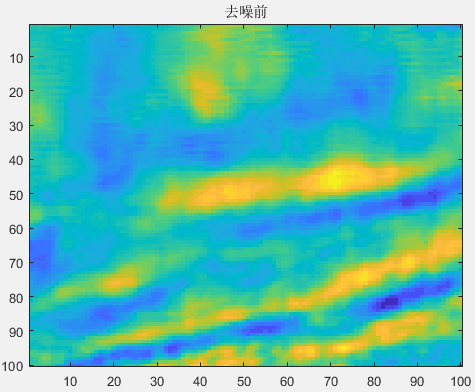处理后：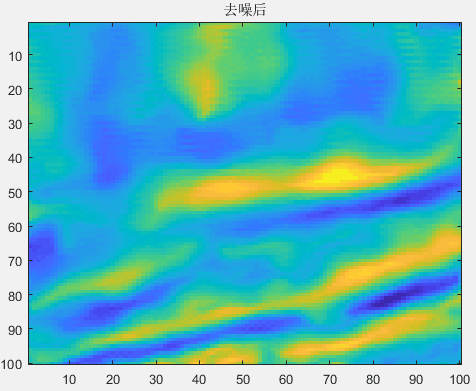差值：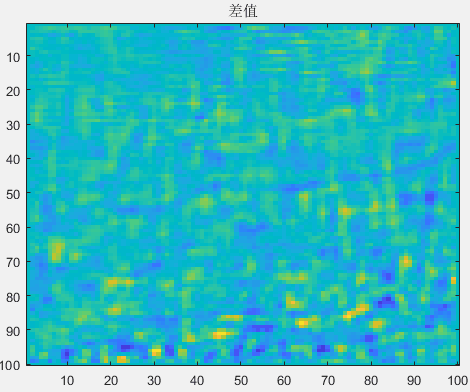展开全文C++
• Kalman 滤波涉及的数学内容比较多，网上有很多讲卡尔曼滤波原理的文章，数学功底欠佳的读者可能看不懂。Sugar 本篇的目标是：用更通俗易懂的方式表达“线性 Kalman 滤波的效果”，让读者不需要太深的数学功底就能...
• 基本去噪滤波器存在的问题：各向同性滤波，比如box filter/Gaussian filter。这类滤波器在能够平滑噪声的影响的同时，也会抹去一些细节，减弱edge在图片中的表现。有时我们需要保留edge的feature，因此有一些人对...
• MATLAB实现导向滤波，提高处理后图像的质量。经过了指导性滤波的处理， 使处理后的图像更加平滑， 除去了噪声的干扰。
• 导向滤波同样是一种平滑滤波算法，其与最小二乘滤波和双边滤波相比，同样是一种具有边缘保持的功能的图形滤波算法，可以用于处理图形噪点较多的图像，而且此种滤波算法与最小二乘滤波和双边滤波相比，有其独特的特点...
• 导向滤波磨皮 原文地址：http://blog.csdn.net/hjimce/article/details/45421299  作者：hjimce 导向滤波磨皮的对应文献为：Guided Image Filtering，这个算法速度极其之快，比其他的保边缘磨皮算法都快...opencv
• 导向滤波磨皮的对应文献为：Guided Image Filtering，这个算法速度极其之快，比其他的保边缘磨皮算法都快，甚至快上好几倍。这个算法最初来自于何明凯的图像去雾算法中，现在已然被应用封装与matlab图像处理函数库中...
• style: plain #guided filter导读 本文为笔者阅读Kaiming He于2013年发表的guided image filtering时的笔记和实验...2.基于matlab的简单实现 论文阅读及算法原理介绍 先给出线性移-变滤波器的基本概念：给出输入...
• 自从何凯明提出导向滤波后，因为其算法的简单性和有效性，该算法得到了广泛的应用，以至于新版的matlab都将其作为标准自带的函数之一了，利用他可以解决的所有的保边滤波器的能解决的问题，比如细节增强、HDR压缩、...
• 作者提出了一种新的梯度域引导图像滤波器，通过将明确的一阶边缘感知约束结合到现有的引导图像滤波器中。...matlab代码实现 转载至：https://blog.csdn.net/majinlei121/article/details/50717777 %主程序fun...
• 前言本文解析四种常见的图像滤波算法，并附上源码。图像滤波是一种非常重要的图像处理技术，现在大火的卷积神经网络其实也是滤波的一种，都是用卷积核去提取图像...目录自适应中值滤波高斯滤波双边滤波导向滤波自适...
• 暗通道先验去雾matlab代码，包括导向滤波代码
• 1暗通道先验，2导向滤波算法。图像去雾 darkchannel MATLAB实现。
• 在图像处理中经常会用到...注：代码是关于导向滤波论文中的源码，大家可以自己下载 function dataVariance = getLocalVariance(srcData, nHeight, nWidth, r) % 计算局部方差 N = boxfilter(ones(nHeight, nWid...
• 一个关于图像去雾的基本算法代码的...暗通道方法采用改进算法，增加了图像采样，提升了计算速度，改进了导向滤波最小二乘的算法使其精细透视率图的效果得到了显著提升。另外附带相应根据上述代码编写的GUI设计的m文件
• 上述伪代码中，I表示导向图像（guided image）,p为输入图像（input image）,q为输出图像（output image），表示均值滤波，r为窗口半径。 ** %-------------------------------------- clc; clear; close all; %% ...
• 上述伪代码中，I表示导向图像（guided image）,p为输入图像（input image）,q为输出图像（output image），表示均值滤波，r为窗口半径。 ** %-------------------------------------- clc; clear; close all; %% ...# 导向滤波matlabmatlab 订阅